Vlookup算什么!Wlookup函数才是万能的!

•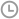发布时间：2019-09-04 16:37:01
• |
• 出处：PDF猫
• |
• 阅读量：177

Wlookup函数

= Wlookup(查找内容，查找值范围,返回值范围,查找模式)

-2 是区间查找

-1 是一对多查找

0 查找最后一个

N 查找第N个符合条件的值

1、查找第1个符合条件的值

=Wlookup(A11,A2:A7,C2:C7)2、从右向左查找

=Wlookup(A11,B2:B7,A2:A7)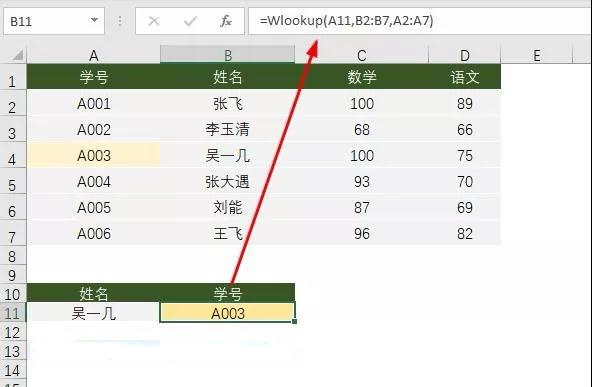3、按行上下查找

=Wlookup(B5,A1:D1,A2:D2)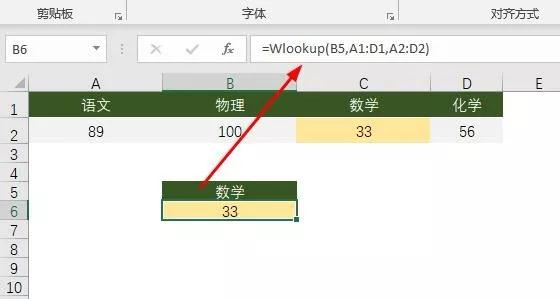4、多条件查找

=Wlookup(A11&B11,A2:A7&B2:B7,D2:D7)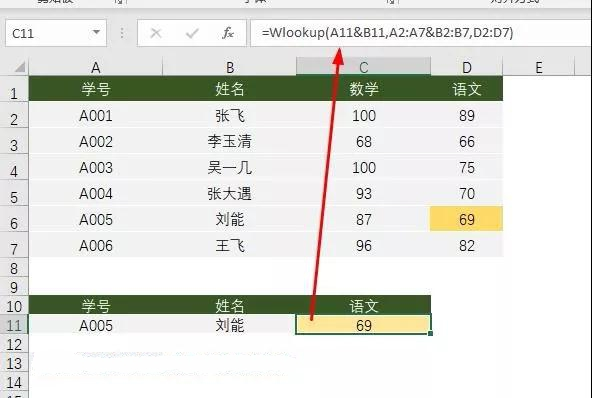5、查找第N个符合条件的值

=Wlookup(A11,B2:B7,C2:C7, N)

=Wlookup(A11,B2:B7,C2:C7, 2)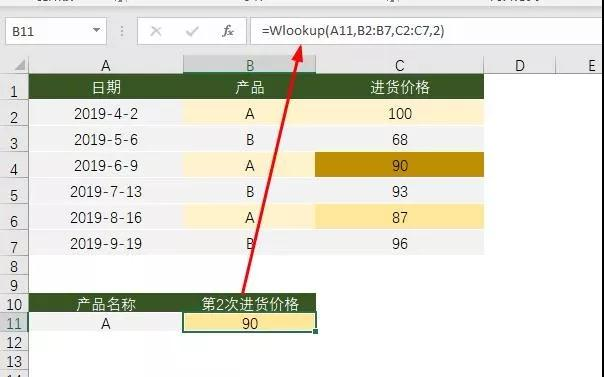6、查找最后一个

=Wlookup(A11,B2:B7,C2:C7,0)7、一对多查找

Wlookup可以同时返回所有符合条件的结果，并用逗号连接。

=Wlookup(A11,B2:B7,C2:C7,-1)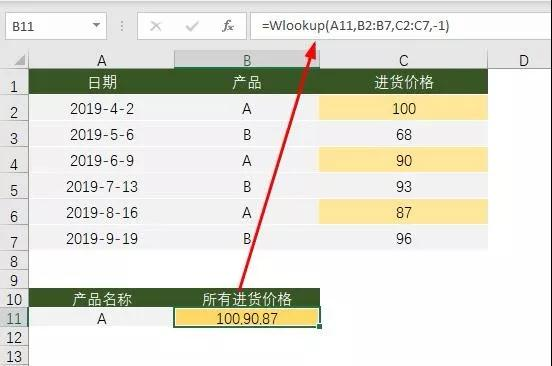8、区间查找

=Wlookup(A11,A2:A7,B2:B7,-2)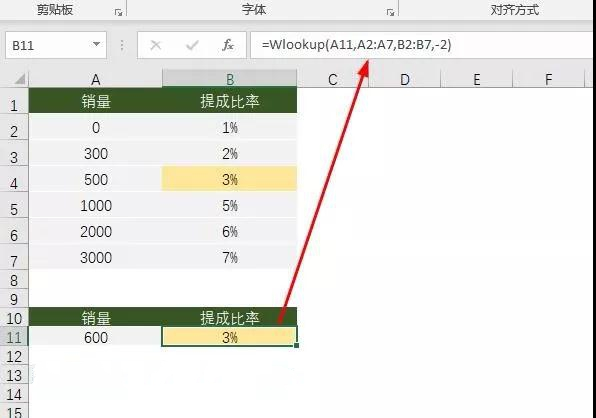9、扩展应用：筛选功能

=IFERROR(Wlookup(\$A\$11,\$B\$2:\$B\$7,A\$2:A\$7,ROW(A1)),"")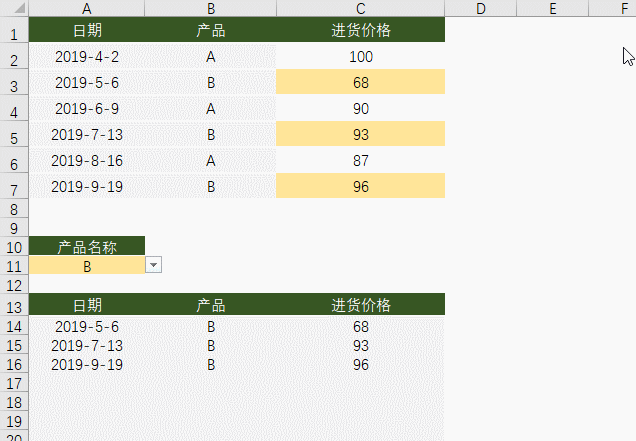Wlookup要想在你的表格中也能使用，需要按下面的步骤操作。

1、按alt+F11(或任一工作表标签右键 - 查看代码)会打开VBE窗口，在窗口中点插入 - 模块。把下面的代码复制粘贴到右侧的空白区域中。Function Wlookup(V, vY, vh, Optional m)

Dim arr, arr1, arr2()

Dim k As Integer

arr = vY

arr1 = vh

If UBound(arr1) = 1 Then

arr1 = Application.Transpose(arr1)

arr = Application.Transpose(arr)

End If

ReDim arr2(1 To 1)

For x = 1 To UBound(arr1)

If arr(x, 1) = V Then

Wlookup = arr1(x, 1)

If IsMissing(m) Then

Exit Function

Else

k = k + 1

ReDim Preserve arr2(1 To k)

arr2(k) = arr1(x, 1)

End If

End If

Next x

If m = 0 Then

Wlookup = arr2(k)

ElseIf m = -1 Then

Wlookup = Join(arr2, ",")

ElseIf m = -2 Then

Wlookup = JS(V, vY, vh)

Else

Wlookup = arr2(m)

End If

End Function

Function JS(J1, R1, R2) '取接近值

Dim Jarr1, Jarr2

Dim x

Jarr1 = R1

Jarr2 = R2

For x = 1 To UBound(Jarr1)

If x + 1 > UBound(Jarr1) Then

JS = Jarr2(x, 1)

Exit Function

ElseIf J1 >= Jarr1(x, 1) And J1 < Jarr1(x + 1, 1) Then

JS = Jarr2(x, 1)

Exit Function

End If

Next x

End Function

2、当前文件另存为“启用宏的工作簿”格式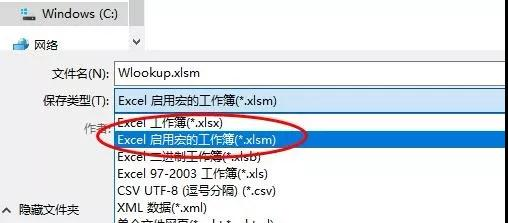PDF猫转换器

PDF猫转换器